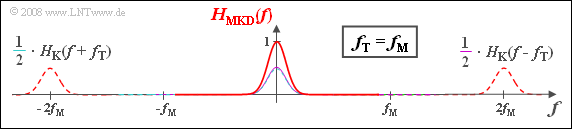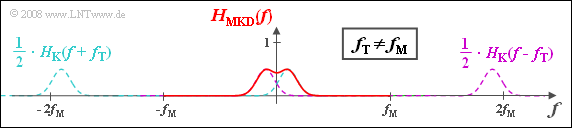# Exercise 1.10Z: Gaussian Band-Pass

For this exercise we assume:

• Binary phase modulation  $\rm (BPSK)$  is used for modulation.
• Demodulation is synchronous in frequency and phase.

For carrier frequency modulated transmission,  the channel frequency response  $H_{\rm K}(f)$  must always be assumed to be a band-pass.  The channel parameters are  e.g.  the center frequency  $f_{\rm M}$  and the bandwidth  $\Delta f_{\rm K}$,  where the center frequency  (German:  "Mittenfrequenz"   ⇒   subscipt:  "M")  $f_{\rm M}$  often coincides with the carrier frequency  (German:  "Trägerfrequenz"   ⇒   subscipt:  "T")  $f_{\rm T}$.

In this exercise we will assume a Gaussian band-pass according to the diagram.  For its frequency response holds:

$$H_{\rm K}(f) = {\rm exp} \left [ - \pi \cdot \left ( \frac {f - f_{\rm M} }{\Delta f_{\rm K}}\right )^2 \right ] +{\rm exp} \left [ - \pi \cdot \left ( \frac {f + f_{\rm M} }{\Delta f_{\rm K}}\right )^2 \right ]$$

For a simpler description,  one often uses the equivalent low-pass  ("TP")  frequency response  $H_{\rm K,TP}(f)$.  This results from  $H_{\rm K}(f)$  by

• truncating the components at negative frequencies,
• shifting the spectrum by  $f_{\rm T}$  to the left.

In the considered example with  $f_{\rm T} = f_{\rm M}$  for the equivalent low-pass frequency response results:

$$H_{\rm K,\hspace{0.04cm} TP}(f) = {\rm e}^ { - \pi \hspace{0.04cm}\cdot \hspace{0.04cm}\left ( {f }/{\Delta f_{\rm K}}\right )^2 }.$$

The corresponding time function  ("inverse Fourier transform")  is:

$$h_{\rm K,\hspace{0.04cm} TP}(t) = \Delta f_{\rm K} \cdot {\rm e}^ { - \pi \hspace{0.04cm}\cdot \hspace{0.04cm}\left ( {\Delta f_{\rm K}} \cdot t \right )^2 }.$$

However,  the frequency response is also suitable for describing a phase-synchronous BPSK system in the low-pass range

$$H_{\rm MKD}(f) = {1}/{2} \cdot \left [ H_{\rm K}(f-f_{\rm T}) + H_{\rm K}(f+f_{\rm T})\right ] ,$$

where  "MKD"  stands for  "modulator – channel (Kanal) – demodulator".  Often - but not always -  $H_{\rm MKD}(f)$  and  $H_{\rm K,TP}(f)$  are identical.

Notes:

### Questions

1

Give the impulse response  $h_{\rm K}(t)$  of the Gaussian band-pass channel.  What is the  (normalized)  value for time  $t = 0$?

 $h_{\rm K}(t)/\Delta f_{\rm K} \ = \$

2

Which statements are valid under the condition  $f_{\rm T} = f_{\rm M}$?

 $H_{\rm K,TP}(f)$  and  $H_{\rm MKD}(f)$  coincide completely. $H_{\rm K,TP}(f)$  and  $H_{\rm MKD}(f)$  are the same for low frequencies. The time function  $h_{\rm K,TP}(t)$  is real. The time function  $h_{\rm MKD}(t)$  is real.

3

Which statements are true under the condition  $f_{\rm T} \neq f_{\rm M}$?

 $H_{\rm K,TP}(f)$  and  $H_{\rm MKD}(f)$  coincide completely. $H_{\rm K,TP}(f)$  and  $H_{\rm MKD}(f)$  are the same for low frequencies. The time function  $h_{\rm K,TP}(t)$  is real. The time function  $h_{\rm MKD}(t)$  is real.

4

What should be true with respect to a smaller bit error probability?

 $f_{\rm M} = f_{\rm T}$, $f_{\rm M} \neq f_{\rm T}$.

### Solution

#### Solution

(1)  For the band-pass frequency response  $H_{\rm K}(f)$  we can write:

$$H_{\rm K}(f) = H_{\rm K,\hspace{0.04cm} TP}(f) \star \big [ \delta (f - f_{\rm M}) + \delta (f + f_{\rm M}) \big ] .$$
• The Fourier inverse transform of the bracket expression yields a cosine function of frequency  $f_{\rm M}$  with amplitude  $2$.
• Thus,  according to the convolution theorem:
$$h_{\rm K}(t) = 2 \cdot \Delta f_{\rm K} \cdot {\rm exp} \left [ - \pi \cdot \left ( {\Delta f_{\rm K}} \cdot t \right )^2 \right ] \cdot \cos(2 \pi f_{\rm M} t ) \hspace{0.3cm} \Rightarrow \hspace{0.3cm}h_{\rm K}(t = 0)/\Delta f_{\rm K} \hspace{0.1cm}\underline {= 2}.$$
• This means:  The low-pass impulse response  $h_{\rm K,\hspace{0.04cm}TP}(t)$  is identical in shape to the envelope of the band-pass impulse response  $h_{\rm K}(t)$,  but twice as large.Resulting baseband frequency response for $f_{\rm T} = f_{\rm M}$

(2)  Statements 2, 3 and 4  are correct:

• The first statement is false because  $H_{\rm MKD}(f)$  also has components around  $\pm 2f_{\rm T}$.
• The time function  $h_{\rm K,\hspace{0.04cm}TP}(t)$  is real according to the given equation.
• The same is true for  $h_{\rm MKD}(t)$  also considering the  $\pm 2f_{\rm T}$  parts,  since  $H_{\rm MKD}(f)$  is an even function with respect  to $f = 0$.
• The diagram shows  $H_{\rm MKD}(f)$,  which also has components around  $\pm 2f_{\rm T}$.  At low frequencies,  $H_{\rm K,\hspace{0.04cm}TP}(f)$  is identical to  $H_{\rm MKD}(f)$.Resulting baseband frequency response for $f_{\rm T} \ne f_{\rm M}$

(3)  Only solution 4 is correct:

• Here $H_{\rm K,\hspace{0.04cm}TP}(f)$ and $H_{\rm MKD}(f)$ differ even at the low frequencies.
• $H_{\rm K,\hspace{0.04cm}TP}(f)$  is a Gaussian function with maximum at  $f_{ε} = f_{\rm M} - f_{\rm T}$.
• Because of this asymmetry,  $h_{\rm K,\hspace{0.04cm}TP}(t)$  is complex.
• In contrast,  $H_{\rm MKD}(f)$  is still an even function with respect to  $f = 0$  with real impulse response  $h_{\rm MKD}(t)$.
• $H_{\rm MKD}(f)$  is composed of two Gaussian functions at  $± f_ε$.

(4)  Correct is of course the  first answer.